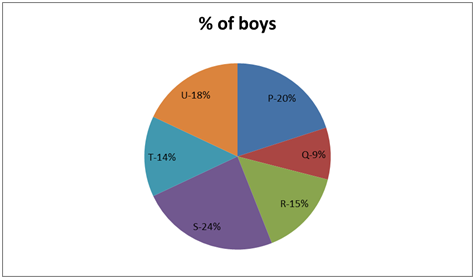# RRB Clerk Prelims Quantitative Aptitude (Day-17)

## Quantitative Aptitude Materials for RRB Clerk

Dear Aspirants, Our IBPS Guide team is providing new series of Quantitative Aptitude Questions for RRB Clerk 2020 Prelims so the aspirants can practice it on a daily basis. These questions are framed by our skilled experts after understanding your needs thoroughly. Aspirants can practice these new series questions daily to familiarize with the exact exam pattern and make your preparation effective.

Start Quiz

Ensure Your Ability Before the Exam Take IBPS RRB Clerk 2020 Prelims Free Mock Test

Application Sums

1) The circumference of a circle is equal to the perimeter of a square. The side of the square is 55 cm. What is double the diameter of the circle?

A) 35 cm

B) 120 cm

C) 140 cm

D) 84 cm

E) 70 cm

2) To complete a work Jeba takes 50% more time than Josh. If together they take 21 days to complete the work, how much time shall Josh take to do it alone?

A) 45   days

B) 40 days

C) 30 days

D) 35 days

E) 25 days

3) A sells a bike to B at a profit of 20%. B sells it to C at a profit of 25%. C sells it at a loss of 10% to D. If D pays Rs.54000 for it, the cost price of the bike for A is

A) Rs.36000

B) Rs.40000

C) Rs.42000

D) Rs.38000

E) Rs.32000

4) If the speed of a car be 62.4 kmph, how many meters would it cover in 15 minutes?

A) 1500 meters

B) 1560 meters

C) 156000 meters

D) 156 meters

E) 15600 meters

5) The ratio of speed of current to that of speed of the boat is 5: 11. If the boat goes downstream in 3 hours 30 minutes, then it will return in

A) 9 hours 20 min

B) 10 hours

C) 8 hours 40 min

D) 6 hours 50 min

E) 7 hours 45 min

Data Interpretation

Study the following information carefully and answer the questions given below.

The pie chart shows the percentage distribution of number of boys in six different schools.

Total number of boys = 24006) If the ratio of the number of boys to number of girls in school “T” is 7: 8. Then find the number of girls in school “T”.

A) 324

B) 384

C) 336

D) 368

E) 372

7) Total number of boys in school “P” is what percent more than total number of boys in school “T”?

A) 33 (1/3) %

B) 66 (2/3) %

C) 38 (4/7) %

D) 42 (6/7) %

E) 50 %

8) Find the average number of boys in school Q, R and S together?

A) 384

B) 354

C) 324

D) 360

E) 408

9) Find the central angle of boys in school “U”?

A) 720

B) 68.20

C) 79.60

D) 57.20

E) 64.80

10) Total number of boys in school “P” is how much more then total number of boys in school “Q”?

A) 330

B) 264

C) 396

D) 363

E) 298

The circumference of a circle is equal to the perimeter of a square = 2πr = 4a

2 * 22/7 * r = 4 * 55

r = 35

Diameter = 2r = 70

Double the diameter of the circle = 2 * 70 = 140

Time ratio between Jeba and Josh = 3: 2

Efficiency ratio between Jeba and Josh = 2: 3

Together efficiency = 5

5 × 21 = 3 × x

Josh work = 35 days

Cost price of A = x

x × 120/100 × 125/100 × 90/100 = 54000

x = Rs.40000

Distance = speed * time

D = 62.4 × 15/60 × 1000

D = 15600 meters

The ratio of speed of current to that of speed boat is 5: 11

From this ratio of speed of upstream = 11 – 5 = 6

Speed of downstream = 11 + 5 = 16

Distance same

16 × 3 (½) = 6 × x

= > x = 9 (1/3) = 9 hours 20 min

Directions (6-10):

Number of boys in school T = 2400 × 14/100 = 336

Ratio between number of boys to number of girls in school “T” = 7: 8

Number of girls in school T = 7x = 8 × 336

Number of girls in school T = 384

Required percentage = (20 – 14)/14 * 100

= 6/14 * 100

= 300/7 = 42 (6/7)% more

Average number of boys in school Q, R and S = (9 + 15 + 24)/3 = 16%

= 16/100 * 2400 = 384

Required angle = x * 100 = 18 * 360

Required angle = 64.80

Total number of boys in school “P” = 20/100 * 2400 = 480

Total number of boys in school “Q” = 9/100 * 2400 = 216

Difference = 480 – 216 = 264

 Check Here to View RRB Clerk Prelims 2020 Quantitative Aptitude Questions Day – 16 Day – 15 Day – 14